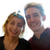0
Fixed

# float4x4 output to float4x4 input converts to float

behealed 7 years ago updated by Freya Holmér (Developer) 7 years ago

Shader Forge converts the float4x4 into a regular float.

Steps to reproduce:

1) Create three "code" nodes.

2) Set one code node to output a Matrix4x4

3) Set the other TWO code nodes to receive a Matrix4x4 input

4) Connect the 4x4 output of the first node, to the 4x4 inputs of the two others

5) Compile, and then manually open the shader to see what it did.

It'll have done this:

```float4x4 Node1 () {
return float4x4(...);
}

float3 Node2( float4x4 IN) {
}

float3 Node2( float4x4 IN) {
}

frag() {
float node_1234 = Node1(); //<-- HERE IS THE PROBLEM, it converts float4x4 to "float".
float3 node_5678 = Node2( node_1234 );
float3 node_5679 = Node3( node_1234 );
}```